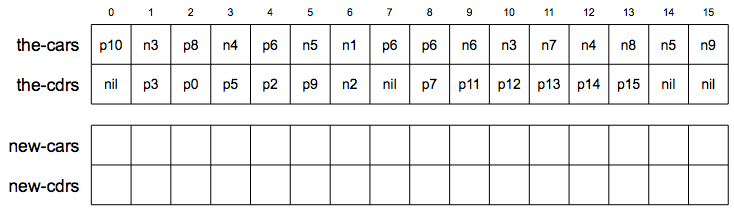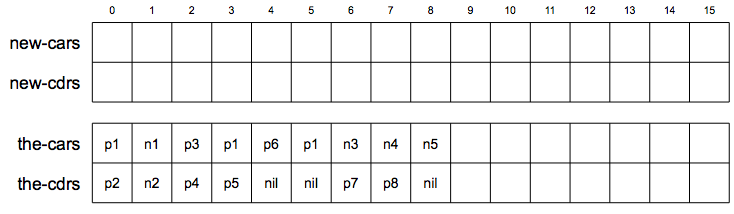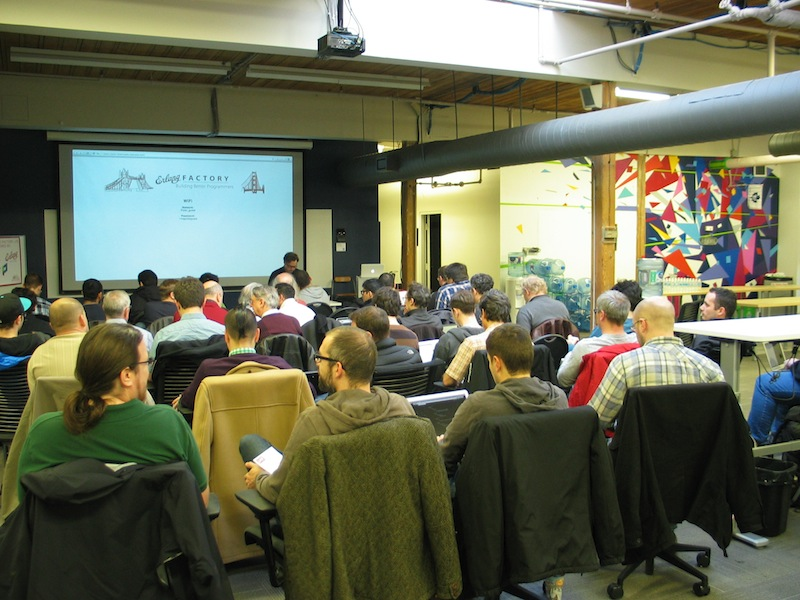• ## GC visualization

While I was working through the chapter 5.3 of SICP, I created small visualization of the stop-and-copy garbage collection algorithm.The content of the root register is a pointer p4 to the list of registers (x y z). The register x points to address 6 where improper list (1 . 2) saved. The register y points to address 8 where list (x x) starts. Finally, the register z points to address 10 where list (3 4 5) starts. Addresses 1, 3, 5, 9, 11, 13, 15 contain garbage.

After we ran the GC algorithm, we got the following memory structure.root now points to address 0, x to 1, y to 3, and z to 6.

• ## Fast Fourier transform in J

In the previous post we discussed the discrete Fourier transform (DFT). Its J implementation was pretty straightforward, and the program looked almost identical to its mathematical definition. In this post, I want to explore how to implement fast Fourier transform (FFT), a recursively defined version of DFT that reduces algorithmic complexity from $O(n^2)$ to $O(N\log{N})$.

## Math

Recall the DFT formula from the previous post

I use a slightly different notation here to make recursive definition easier to understand.

We assume that N is a power of 2. Using basic arithmetic and properties of complex exponentials, we can derive the formula for the DFT of order 2N in terms of two DFTs of order N

where $m=0,\dots,N-1$. The base case, when $N=1$, is

i.e. the identity function.

• ## Discrete Fourier transform in J

The Fourier analysis in general and the Fourier transform in particular has always been one of my favourite topics in mathematics. When I started learning the J programming language I thought that implementing the discrete Fourier transform (DFT) in J must be really elegant. One rainy weekend I finally found time to do that, and this post is a result of my experiments.

## Math

The definition of one-dimensional DFT is pretty simple. It is just a function that maps a given vector f of length N to a vector F of the same length by the following formula

To implement this formula the only thing we need is basic arithmetic for complex numbers. Not only does J provide such an arithmetic, it has special code for complex exponentiation, which makes the implementation much easier than it would be in other languages.

Before we start coding let’s rewrite the formula in terms of matrix multiplication so that we can utilize this feature of J. Let $\omega$ be the primitive Nth root of unity $\omega = e^{2\pi i/N}$, then the DFT formula becomes

where f is an input vector and $\Omega$ is a symmetric matrix $(\omega^{-mn})$ with $m,n=0,\dots,N-1$.

• ## Erlang Factory Lite 2013

For the last four months I’ve been actively involved in organizing EFL in Toronto. Now when the conference is over I want to take a few minutes to express my appreciation to all the people who made it happen.My big thank you goes to (in alphabetical order)

• Carlo Barrettara, Wioletta Dec, Michael DiBernardo, Monika Jarzyna, Michael Russo, Dann Toliver
• All speakers: Louis-Philippe Gauthier, Fred Hebert, Christopher Meiklejohn, Igor Ostaptchenko, Yurii Rashkovskii, Tom Santero, Garrett Smith
• All attendees
• My family

Thank you all! Without you this conference wouldn’t be possible.

• ## 12PM bug in Java

I’ve recently hit on a nasty bug in Java. It sits in Date class and shows up only at noon! I’m going to demonstrate it using Groovy shell, but you can reproduce it in plain Java environment too.

So far so good.

I chose a specific formatter on line 1 to make the bug even more evident. With this formatter lines 5, 8, and 11 must be identical on my machine, and they are in this example. The assertion on line 13 also proves the equality.

Now let’s change the example date to one hour earlier

Lines 2, 5, and 8 are not identical any more. The String representation of the date is 12 hours off.

To make sure the problem is in toString and not in parse, let’s format the date using formatter

Looks good. The problem is in toString indeed. Or is it?

Let’s parse 13 o’clock date and 12 o’clock date. The difference between them should be 1 hour. In reality

Wow, the problem is actually in parse. Then how come the format returned the correct value? That’s still a mystery to me.

I’m actually quite surprised that this bug survived through JDK 1.7.0_09, and neither Sun nor Oracle hasn’t fixed it yet.